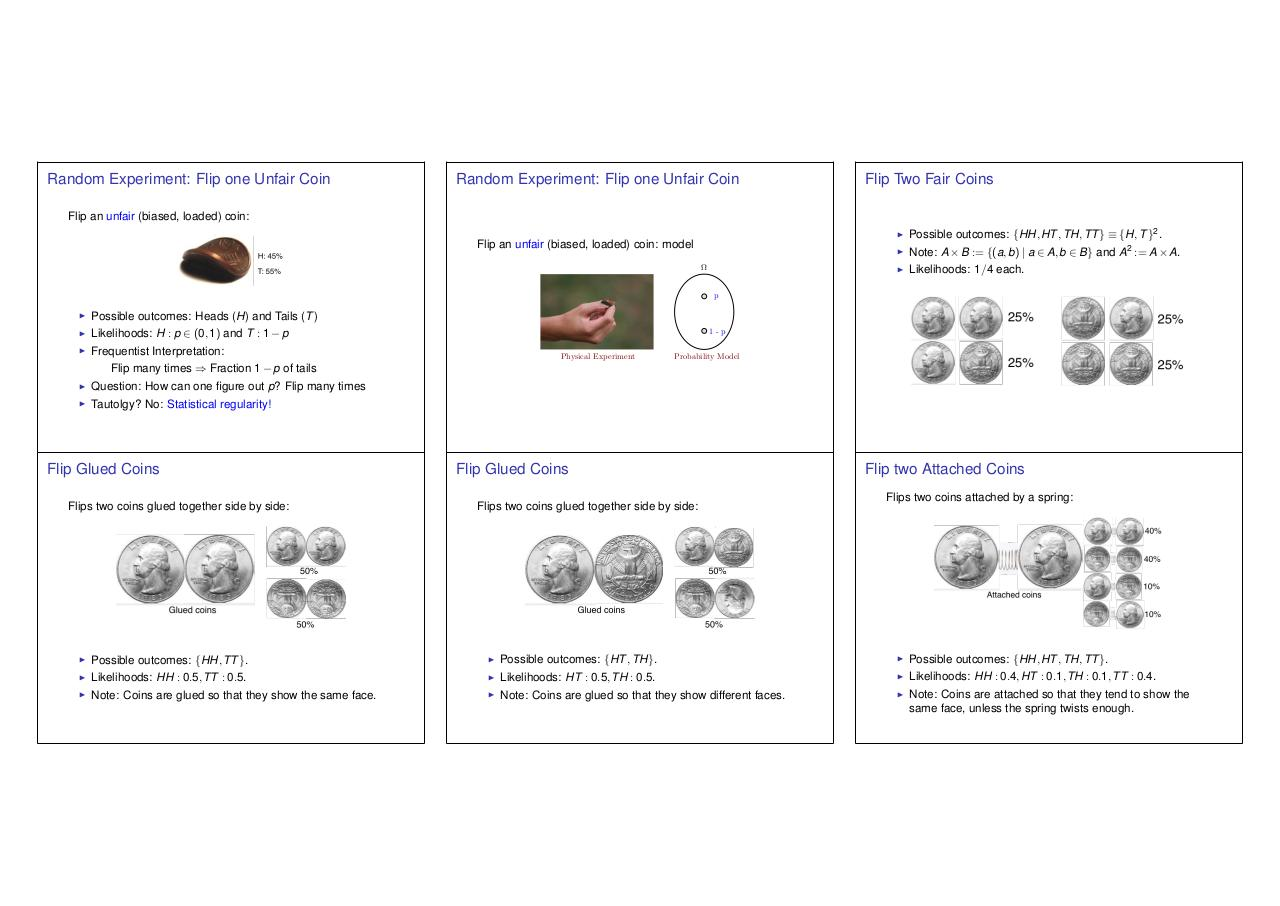# lec 15.6up.pdfPage 1 2 3 4 5 6 7

#### Text preview

Random Experiment: Flip one Unfair Coin

Random Experiment: Flip one Unfair Coin

Flip Two Fair Coins

Flip an unfair (biased, loaded) coin:
I

Flip an unfair (biased, loaded) coin: model

I
I

Possible outcomes: {HH, HT , TH, TT } ⌘ {H, T }2 .

Note: A ⇥ B := {(a, b) | a 2 A, b 2 B} and A2 := A ⇥ A.
Likelihoods: 1/4 each.

p

I

Possible outcomes: Heads (H) and Tails (T )

I

Likelihoods: H : p 2 (0, 1) and T : 1

I

p

Frequentist Interpretation:

Flip many times ) Fraction 1

Physical Experiment

Question: How can one figure out p? Flip many times

I

Tautolgy? No: Statistical regularity!

Flip Glued Coins
Flips two coins glued together side by side:

I
I

Probability Model

p of tails

I

I

1-p

Flip Glued Coins
Flips two coins glued together side by side:

Possible outcomes: {HH, TT }.

I

Likelihoods: HH : 0.5, TT : 0.5.

I

Note: Coins are glued so that they show the same face.

I

Flip two Attached Coins
Flips two coins attached by a spring:

Possible outcomes: {HT , TH}.

I

Likelihoods: HT : 0.5, TH : 0.5.

I

Note: Coins are glued so that they show different faces.

I

Possible outcomes: {HH, HT , TH, TT }.

Likelihoods: HH : 0.4, HT : 0.1, TH : 0.1, TT : 0.4.
Note: Coins are attached so that they tend to show the
same face, unless the spring twists enough.